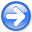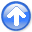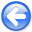Fast log-decon with a quasi-Newton solver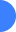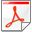Next: Bibliography Up: APPENDIX Previous: The limited-memory BFGS method

## An efficient algorithm for solving nonlinear problems

The solver works as follows:
1. Choose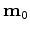,,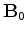. Set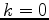.
2. Compute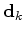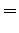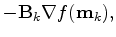(8)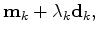(9)

where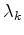meets the Wolfe conditions.
3. Let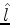=min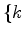,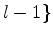. Update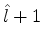times using the pairs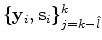, i.e., let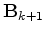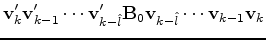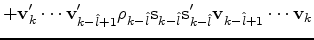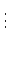(10)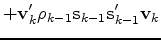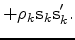(11)

4. Setand go to 2 if the residual power is not small enough.
The updateis not formed explicitly; instead we compute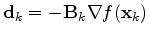with an iterative formula Nocedal (1980). Liu and Nocedal (1989) propose scaling the initial symmetric positive definiteat each iteration as follows: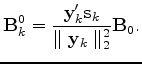(12)

This scaling greatly improves the performances of the method. Liu and Nocedal (1989) show that the storage limit for large-scale problems has little effects. A common choice foris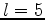. In practice, the initial guessfor the Hessian is the identity matrix; then it might be scaled as proposed in equation (12). The nonlinear solver as detailed in the previous algorithm converges to a local minimizer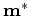of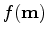.Fast log-decon with a quasi-Newton solverNext: Bibliography Up: APPENDIX Previous: The limited-memory BFGS method

2012-10-29